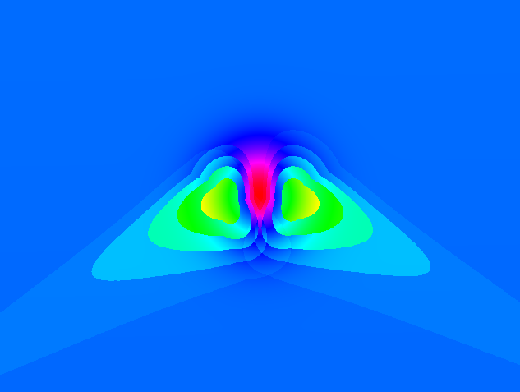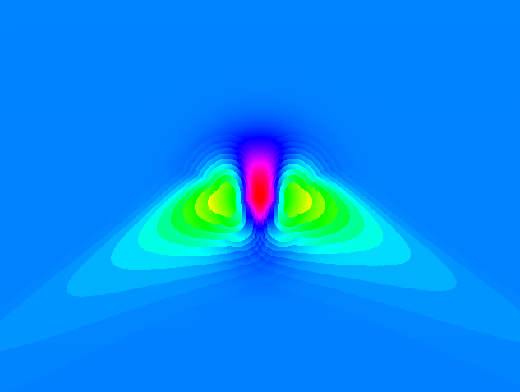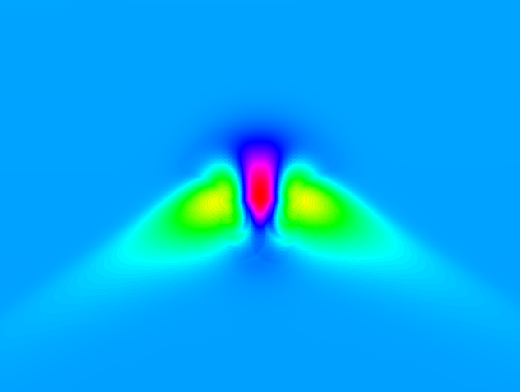# Difference between revisions of "R.sun"

## Tips

The speed of r.sun is much higher if r.horizon is used first and the resulting maps are given as input to r.sun. Background: the horizon needs to be computed only one time before, not in every step within r.sun. See the example at the end of the r.sun help page.

### Testing

Create an artificial surface containing a Gaussian mound:

```r.surf.volcano out=gauss method=gaussian kurtosis=1
```

### Time step

The following three images demonstrate the effects of using different time step parameters.

```r.sun -s elevin="gauss" glob_rad="rad.global.30minT" day=180 step=0.5
```Default 30 minute time step over a Gaussian mound.

```r.sun -s elevin="gauss" glob_rad="rad.global.15minT" day=180 step=0.25
```15 minute time step over a Gaussian mound.

```r.sun -s elevin="gauss" glob_rad="rad.global.03minT" day=180 step=0.05
```3 minute time step over a Gaussian mound.

The 3 minute time step takes roughly ten times as long to run as the 30 minute timestep.

## ToDo

• Add support for OpenMP parallelization